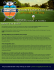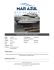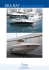# Arbitrary 3D Resolution Discrete Ray Tracing of Implicit Surfaces

## Transcription

Arbitrary 3D Resolution Discrete Ray Tracing of Implicit Surfaces
```Arbitrary 3D Resolution Discrete Ray Tracing of
Implicit Surfaces
Nilo Stolte
École de Technologie Supérieure, 1100 rue Notre-Dame Ouest, Montréal H30 1K3, Canada
[email protected]
Abstract. A new approach to ray tracing implicit surfaces based on recursive
space subdivision is presented in this paper. Interval arithmetic, already used to
calculate intersections in ray tracing and ray casting (numerically or subdividing
1D or 2D spaces), is now used here to implement a ray tracing based on reliable
rays traversals into a potentially infinite octree-like subdivided space, eliminating
explicit intersections. Novel, robust and efficient algorithms for ray voxelization
and BSP octant ordering are used to recursively traverse rays through the space.
Implicit surfaces are robustly voxelized and hierarchically stored into an octree
to a certain given level. During rendering, the subdivision based voxelization of
surfaces and rays continues further down until a resolution near the discrete domain of the floating point numbers is acquired. To guarantee robustness of the
ray voxelization, interval arithmetic with calculations performed under appropriate rounding modes in Pentium-4 x87 and SSE2 FPUs respectively is applied.
The major advantage is that the traversal algorithm is guaranteed to find reliable
intersections between the rays and the scene without any explicit intersection calculation, solving a known precision problem of the ray traversal in a previous
approach, used here for comparison. The precision of the traversal can be arbitrarily increased within the limitation of the floating point representation.
1
Introduction
Ray tracing has been relying intensively on rays-objects intersections  which have
been persistently imputed as the cause of its low efficiency. Acceleration techniques
have been proposed to reduce in one way or another the number of intersection calculations. Some are based on space subdivision in which explicit ray-object intersections are
bypassed by traversing rays through the subdivided space. Nonetheless, the ray traversal
itself might rely on intersections between rays and bounding volumes [2, 3] or between
rays and discrete subspaces where the scene was previously voxelized [4–7]. Octrees [8,
4, 6, 5, 7, 9] have been proved to have great advantages for space decomposition in these
techniques, since empty regions can be efficiently skipped. However, their advantages
have been considerably hindered by the fact that the intersection calculations between
rays and the boundaries of the regions traversed are not only inefficient but also unreliable . Sometimes, these intersection calculations were implicitly or exclusively
accomplished by incremental algorithms [8, 10, 7, 9]. Even though incremental algorithms are more efficient than direct intersection calculations they lack precision and
are not reliable. As the discrete traversal advances, the mismatch between the continuous ray and the discrete counterpart considerably increases due to the lack of precision,
thus parts of the scene would have the tendency to disappear at the end of the traversal
since what the discrete ray intersects may be different from what the floating point ray
intersects. Evidently, if the discrete traversal is done in low resolution spaces, as it is
the case in most acceleration algorithms, the problem is less noticeable. However, the
problem is particularly worse when considerably huge discrete spaces are traversed.
Prior to the algorithm presented in section 2, robust ray traversals in discrete spaces
have not yet been proposed to solve the problem, although there have been several
robust techniques presented such as Lipshitz conditions or interval arithmetic. Interval
arithmetic was introduced by Moore [11, 12] and by Duff and Snyder [13, 14] into computer graphics. Since the problem of rounding errors can be very serious as seen in ,
the search for reliable algorithms for rendering is of quite significant importance. Although interval arithmetic has been used in several ray casting approaches [16, 14], the
algorithm in section 2 is the first complete solution of an interval arithmetic based ray
tracing with 3D space subdivision using discrete ray traversal. Kalra and Barr’s ray tracing in  adopted a guaranteed ray intersection technique, that could not be considered
a reliable solution for ray traversal. Their ray tracing  used Lipshitz conditions to
voxelize implicit surfaces. However, explicit intersections between rays and octants as
well as between rays and objects were still calculated. Duff’s interval arithmetic ray
casting  is robust, but it works in the image space and applies the perspective into
the surface equations, thus being not compatible with space subdivision techniques.
In this article, a new approach is shown in which the traversal is done by voxelizing implicitly represented rays using the same technique to voxelize implicit surfaces.
Surfaces and rays are simultaneously voxelized to avoid all explicit intersection calculations, between rays and objects as well as between rays and octants. The correlation
between the discrete ray and the continuous ray is solved here because they are exactly
the same. In this sense this approach resembles a discrete ray tracing [10, 9]. The basic
differences in our approach are: (1) the voxelization of surfaces and rays are robust due
to the use of interval arithmetic; (2) spatial resolution is much higher, allowing reaching
the discrete domain of floating point numbers as proposed in ; (3) the scene is voxelized to a lower resolution into the octree as in  but during rendering the voxelization
of surfaces and rays continues on the fly until a given precision is reached. Even though
voxelization plays a crucial role, methods that do not ensure robustness [19–21] cannot
be used in our context. By chance, implicit surfaces can be voxelized robustly [22, 14,
13, 23, 24] and a huge variety of forms and shapes can be defined implicitly.
Robust voxelization of implicit surfaces is generally implemented using spatial recursive subdivision [22, 14, 23, 24]. The methods are all conservative, though. Thus,
spurious voxels might show up, depending on the surface, the voxelization method and
how it is implemented. Although Lipshitz conditions can also be used to voxelize implicit surfaces , interval arithmetic is preferred in our approach because it is shown
to be more efficient and more reliable . To avoid the spurious regions, the implicit
function describing the surface is evaluated at the eight vertices of the octants to verify
if there is a change in sign when the last level is reached. Since it is only done at the
last level of the subdivision, the robustness throughout the process is guaranteed, but
not in  because it is performed at each octant before testing the Lipschitz condition.
During the recursive subdivision at rendering time the order in which the octants are
to be traversed is important. Our innovative BSP ordering algorithm ensures robustness
#define NL
30
bool intersectionFound = false;
Point intersectedPoint;
int signDir = (dz<0)2+(dy<0)1+(dx<0);
bool Traversal (octant, level) {
if (intersectionFound) return true;
if (level == NL) {
Object obj = (surface contained in octant);
if (!IsoValueTest(obj, octant)) {
if (!PartialDifferentialTest(obj, octant))
return false;
}
intersectionFound = true;
intersectedPoint = mid;
return true;
}
if (!(ray passes through octant)) return false;
int izyx = ((z0 > zm ) 2) + ((y0 > ym ) 1) + (x0 > xm );
int iaux = izyx xor signDir;
for (i=0; i<8; i++) {
if (iaux and i) continue; /* Octant elimination */
int idx = izyx xor i; /* BSP ordering */
suboct = octant[idx];
if (!(r passes through suboct)) continue;
if (suboct contains a part of a surface)
if (Traversal (suboct, level+1)) break;
}
}
return intersectionFound;
Fig. 1. Octree ray traversal algorithm
in this process too. In this algorithm only the starting point of the ray and the middle
point of the octant are required. The middle point is always an integer-like number that
is produced by the addition of a power of two, half of the length of the octant plus the
coordinate of the octant. This is guaranteed to avoid carry propagation, thus ensuring
robustness as well as exactness. This calculation is always exact provided the half length
of the octant is not smaller than 1 Ulp (unit in the last place) .
2
2.1
New octree ray traversal algorithm
Notations & definitions
Ray The notation for the ray equation starting at (x0 , y0 , z0 ) and with (dx, dy, dz) as
its the direction vector is as follows:

 x = x0 + t· dx
y = y0 + t· dy
(1)

z = z0 + t· dz
Scene The scene is contained in an axis-aligned cube defined as the bounding box of all
the surfaces. One of the cube’s vertices is located at the origin (0, 0, 0) and all the other
ones have zero or positive coordinates. All the objects (surfaces) are defined within this
cube, and previously voxelized using space subdivision.
Octant & splitting planes The space subdivision starts splitting the scene into eight
equal sized cubes, and each of these cubes is called an octant. An octant can be viewed
as three intervals, each one along its respective coordinate axis:
[xc , Xc ] [yc , Yc ] [zc , Zc ]
(2)
where (xc , yc , zc ), the vertex with lowest coordinates, is regarded as the coordinate of
the octant, and (Xc , Yc , Zc ) is the vertex with highest coordinates. The subdivision of
an octant is performed along the three axis-aligned splitting planes passing through the
middle point of the octant, that is (xm , ym , zm ) = (xc +l/2, yc +l/2, zc +l/2), where l is
the size of the octant. The splitting planes equations are then x = xm , y = ym and z = zm .
The eight subdivided octants (sub-octants) are stored in memory along an order
determined by their relative locations in respect to the splitting planes, with indices
from 0 to 7. Actually each index consists of three bits, each corresponding to an axis.
Each bit is set if the sub-octant lies on the positive side of the corresponding splitting
plane, otherwise it is zero.
2.2
Algorithm overview
The appealing idea of using discrete space resolutions so high to be able to reproduce
the discrete domain of the floating point numbers to guarantee robustness  is for
the first time implemented in this article. Moreover, the techniques in  to robustly
voxelize different kinds of implicit surfaces are used as a basis in our approach to ray
tracing. A conventional ray tracer is used here, while the intersection calculation is
replaced by our new octree ray traversal algorithm.
During subdivision, each octant that might contain a part of a surface is further
subdivided and so forth. Once a certain level is reached the octant is considered a leaf
node or voxel and stored in an octree. During rendering time, the basic idea is to traverse
each ray through the octree to find the first voxel containing a part of an object in the
scene, which is in appearance similar to the work of Glassner  or Gargantini .
The fundamental difference between their approaches is that Glassner uses a linear
different from their approaches in two aspects: (1) no explicit intersections between rays
and octants neither between rays and objects are ever calculated; (2) the spatial recursive
subdivision continues further down after the first voxel is found. This subdivision is
done in the same way as the recursive voxelization, however, it is done on the fly and
nothing needs to be stored, since rays and surfaces are voxelized concurrently. For each
octant the eight sub-octants are sorted in the order that they might be traversed by
the ray, and tested to verify if they are indeed traversed by the ray. This process is
summarized in the pseudo code of Fig. 1. The IsoValueTest and PartialDifferentialTest
appearing in Fig. 1 are discussed in section 2.5.
In the implementations of Glassner’s  and Gargantini’s  approaches explicit intersection calculation is performed when traversing the subdivided space, which cannot
guarantee robustness. Our approach is significantly more reliable since the ray voxelization (see section 2.4) is robust and the BSP ordering scheme relies on calculations
not affected by rounding errors. The traversal continues to recursively descend in the
subdivided space whenever octants that satisfy the following three conditions are found:
0
1
(a) x0 < xm
x
(b) x0 > xm
xm
(a) Natural order preserved (0-1) (b) Natural order reversed (1-0)
Fig. 2. Illustration of the BSP ordering technique
1. The ray passes through the octant. This is tested using our new ray voxelization
algorithm (see section 2.4).
2. The octant contains a part of a surface in the scene. When the traversal is within the
octree, it is easily tested by verifying the data stored in the octree. When the traversal goes beyond the octree resolution, the implicit function inclusion function 
used to voxelize the surface is reused to check this condition.
3. The octant is the nearest to (x0 , y0 , z0 ) satisfying conditions 1 and 2. This is ensured
using the BSP ordering technique (see section 2.3).
The traversal continues until an octant is finally found after descending NL levels in
the subdivided space, where NL is the preset maximum traversal level, otherwise it is
assumed that there is no intersection between the ray and the scene. The intersection
point is then considered to be (xm , ym , zm ). The precision obtained is dictated by the
maximum distance between this
√ point and the real intersection, that is, half of the octant diagonal length, which is 3 · 2−NL−1 . It can be seen that better precision can be
obtained by increasing NL. For the IEEE 754 double precision numbers, the maximum
value of NL is 53, which is the number of bits in the significand including the hidden 1,
a binary digit always set to 1 except in special cases, which is not explicitly represented.
2.3
Enhanced BSP octant ordering
The BSP ordering technique is shown in Fig. 1. It is based on the relative location of
the starting point of the ray in respect to the three splitting planes. This information is
stored into the variable izyx as illustrated in Fig. 1. The variable i stores the indexes of
the octants as they appear in the memory, but these indexes are not in the front to back
order as required in our ray traversal. As illustrated in Fig. 2, the memory voxel order
is preserved in certain cases; otherwise it is reversed. Moreover, if the ray does not pass
through a splitting plane, the four sub-octants at the opposite side of the splitting plane
in respect to the starting point of the ray will never be traversed and are ignored. The
efficiency of these two calculations comes from their extreme simplicity and reduced
number of instructions.
2.4
Novel robust ray voxelization algorithm
Ray inclusion function Our method to test whether a ray passes through an octant is
based on the implicit form of the ray. After eliminating the t variable in (1) and applying
a common factor |dx|·|dy|·|dz|, the implicit representation of the ray is obtained:
cx·(x − x0 ) = cy·(y − y0 ) = cz·(z − z0 )
where
(3)
cx = sign(dx) · |dy| · |dz|
cy = sign(dy) · |dz| · |dx|
cz = sign(dz) · |dx| · |dy|
Actually, each one of the three parts of (3) is the multiplication of t in (1) by
|dx|·|dy|·|dz|. The sign(dx), sign(dy) and sign(dz) ensure that the values obtained from
(3) have the same sign as the real t in (1). Replacing the variables x, y and z in equation
(3) by the three intervals in (2) produces one interval in each of the three parts of (3), as
shown in (4).
[ fx (xc ), fx (Xc ) ] = [ cx · (xc − x0 ), cx · (Xc − x0 ) ]
[ fy (yc ), fy (Yc ) ] = [ cy · (yc − y0 ), cy · (Yc − y0 ) ]
[ fz (zc ), fz (Zc ) ] = [ cz · (zc − z0 ), cz · (Zc − z0 ) ]
(4)
As can be seen, the ray passes through the octant only if these three intervals overlap
and this condition is what is used to test if a ray passes through an octant.
Using correct rounding modes The approach still suffers from rounding errors caused
by the multiplications. The precision of the traversal is determined by the number of
subdivision levels, each further subdivision corresponding to an additional bit of precision. In experiments where subdivision levels are close to the limits of floating point
double precision (e.g. 50 levels), some octants are missed by rays that pass very close to
octants’ edges or vertices. In this case the two bounds of the octants in each axis differ
only in the last few bits of the significand. Consequently, after applying them to (3) the
resulting intervals are more prone to rounding errors. To guarantee robustness of this
calculation, interval arithmetic with appropriate rounding modes is applied. The calculation in (4) is done with two rounding modes, towards ‘-∞’ and ‘+∞’, to the lower and
upper bounds respectively. Consequently when the three intervals obtained with correct
rounding modes do not overlap, it is assured that the ray does not pass through the octant. Moreover, we consider that there is no overlap when a lower bound of an interval
is equal to the upper bound of another, since the ray in this case passes through neither
one of them. To avoid penalties in performance normally involved in frequent change of
rounding modes, each bound is calculated in a different floating point unit (FPU) preset
with the required rounding mode. The Pentium-4 allows executing 2 double precision
operations in SSE2 FPU and one double precision operation in the normal x87 FPU
with independent rounding modes.
Optimizations The actual implementation of this algorithm differs from what is described in Fig. 1 due to optimization reasons. The number of operations shown in section 2.6 takes these optimizations into account. In Fig. 1, the test to verify if a ray passes
double tx = { xc , xm , xm , Xc };
/* calculate array tx*/
int itx = (signDir and 1)1;
/* but for the other two axes it would be: */
/* int itx = (signDir and 2); → y axis */
/* int itx = (signDir and 4)1; → z axis */
tx[itx] = fx− (tx[itx]);
tx[itx+1] = fx− (tx[itx+1]);
tx[itx xor 2] = fx+ (tx[itx xor 2]);
tx[(itx xor 2)+1] = fx+ (tx[(itx xor 2)+1]);
/* retrieving corresponding interval from tx */
/* idx is the index of the sub-octant as defined in Fig. 1 */
int ix = idx and 1;
/* but for the other two axes it would be: */
/* int ix = (idx and 2)1; → y axis */
/* int ix = (idx and 4)2; → z axis */
double lbx = tx[ix+itx];
double ubx = tx[ix+(itx xor 2)];
Fig. 3. Storing and retrieving the interval bounds with correct rounding modes without testing to
avoid stalling the processor pipeline
through an octant is carried out once for the octant itself and once for each of its suboctants, thus giving rise to 9 tests involving the evaluation of 27 intervals. Since they
share bounds with each other, only 3 intervals along each axis are really considered for
testing all the 9 octants, so only 9 of the 27 intervals are indeed calculated. To avoid
these repetitions all the eight sub-octants and their parent octant are tested together.
What remains to be elucidated is the selection of the correct rounding mode for each
bound of an interval. For the x coordinate, the following three intervals, [xc , Xc ], [xc , xm ]
and [xm , Xc ] will be applied to the implicit ray equation (3), and three resulting intervals
will be obtained. However, the proper rounding modes to guarantee numerical robustness depend on the direction of the ray along the x axis (dx). In the case that dx >0, the
resulting intervals are
[ fx− (xc ), fx+ (Xc )], [ fx− (xc ), fx+ (xm )], [ fx− (xm ), fx+ (Xc )]
where fx− () and fx+ () represent the calculation of fx () in (4) using rounding modes
towards ‘-∞’ and ‘+∞’ respectively. When dx < 0, the resulting intervals will be
[ fx− (Xc ), fx+ (xc )], [ fx− (Xc ), fx+ (xm )], [ fx− (xm ), fx+ (xc )]
Evidently xc and Xc are calculated only once under different rounding modes, and
xm is always calculated twice, each one under a different rounding mode respectively.
Therefore, an array of four elements tx is used to represent the three resulting intervals. When dx >0, tx and tx are calculated with rounding mode ‘towards -∞’,
and tx and tx are calculated with rounding mode ‘towards +∞’; or vice versa when
dx< 0. The same logic is used along y and z axis. To avoid tests and branch instructions
that may stall the processor pipeline, the indices of the elements in the arrays to store
the values calculated under each rounding mode are automatically selected. A similar
scheme is used to retrieve the correct lower and upper bounds stored in tx array for each
sub-octant. The procedure is shown in Fig. 3.
2.5
Partial differential test
The robustness of interval arithmetic can guarantee that no parts of the surfaces were
omitted during voxelization/traversal. On the other hand, however, it cannot guarantee
that each leaf voxel really contains a part of a surface, thus resulting in overestimations
during the voxelization/traversal process. To evaluate and reduce these overestimations,
two algorithms as proposed in  are applied on the final octant and the surface contained in it. The first one calculates the eight iso-values of the function corresponding
to the surface at the eight corners of the octant, and checks if there is a change in sign.
This algorithm may suffer from rounding errors as suggested in , since it directly
uses the surface equation. In some cases, these rounding errors will possibly be added
to the half of the octant diagonal length error described at the end of section 2.2. Even
though it is possible to solve the problem, the reliability at the leaf level is already quite
high; therefore, the use of this algorithm is acceptable.
bool PartialDifferentialTest (Surface, Octant) {
f (x, y, z) : implicit function corresponding to Surface;
DFX(X), DFY (Y ), DFZ(Z) :
inclusion functions of ∂∂xf , ∂∂yf and ∂∂zf ;
X, Y , Z : the three intervals corresponding to Octant;
DX, DY , DZ : three intervals;
DX = DFX(X); DY = DFY (Y ); DZ = DFZ(Z);
return ((0 ∈ DX) k (0 ∈ DY ) k (0 ∈ DZ))
}
Fig. 4. Partial differential test of an octant
If there is a change in sign, the octant is considered to be correct at the given precision, otherwise the second algorithm will be applied, which examines the monotonicity
of the implicit function as shown in Fig. 4. If the partial differential test returns true, the
function is monotonic within the x, y, and z range of the octant, thus the function has
no zeroes within the octant, so the octant is ignored and the traversal continues. If the
test returns false, the traversal stops, despite that in very special cases, this octant may
not contain a part of a surface. This case does not occur in any surface ray traced in this
paper; however, it needs to be considered in future work.
2.6
Comparison with Gargantini’s algorithm
Octree traversal algorithms, such as Gargantini’s , calculate intersections between
the ray and the octants it traverses. Therefore, the traversal algorithm in section 2.4 is
compared with Gargantini’s. The rest of the program is exactly the same.
Gargantini’s algorithm exploits the fact that a ray can pass through at most four
sub-octants in an octant. Assuming that the entry and exit points of a ray into an octant
are known, intersections between the ray and all three subdivision mid-planes are then
calculated using (1). Only the m (m ≤ 3) intersected points that are within the octant are
retained and sorted in ascending order according to their corresponding t values, resulting in m + 1 ray segments. The lower and upper bounds of each segment correspond to
the entry and exit points of the ray into one sub-octant, thus the sub-octants traversed
by the ray are obtained in the correct order. The technique above was described in .
Due to differences between the octants’ structures, the implementation here has some
variations in respect to . Here, an octant only contains an array of pointers to its suboctants. After the index of a sub-octant is known, its coordinates and then its middle
point need to be calculated. In  only the indices of the sub-octants are needed, since
their coordinates are not used, and the octant structure already contains the middle point
coordinates. Because of the variations in design, the number of operations of Gargantini’s algorithm contains more floating point additions and bit operations than what was
claimed in . The extra data cannot be stored in our case since our traversal is also
done on the fly as well as in the octree, while in  it is limited to the octree.
The advantage of the traversal algorithm in  is that it eliminates all the suboctants that are not traversed by the ray. Comparatively, the BSP ordering and auxiliary
techniques in our algorithm can only partially eliminate them, whereas the remaining
ones are still tested by the ray voxelization algorithm in section 2.4. However, as can be
Gargantini’s, and is just slightly slower after performing the calculations using robust
interval arithmetic with different rounding modes. This is due to the high efficiency of
our BSP ordering and ray voxelization techniques.
If the precision/resolution is not too high (e.g. 30 levels), the traversals in both Gargantini’s and our approach are exactly the same for the scenes tested. However, when
subdivision levels become close to the limits of the floating point precision the two traversals start to mismatch. To verify which traversal is correct, a 128 bits precision binary
floating point arithmetic package was used. A verification program using this package
calculates the intersections between a ray and each sub-octant of a traversed octant,
giving the sub-octants indices in the order they are traversed. In all the scenes tested
our approach applying interval arithmetic with correct rounding modes (with SSE2)
always exhibited traversals identical to the ones obtained by the verification program.
Gargantini pointed out in  the two cases when the errors may occur, and described
a method to avoid the choice of incorrect octant under certain conditions restricted to
ray casting. However, the method is not a complete solution for all cases, and can only
eliminate a part of the errors. Comparatively, after applying interval arithmetic with
correct rounding modes, the algorithm described in section 2.4 can guarantee that an
octant will never be missed without much effect in the performance, thus solving the
problem in Gargantini’s algorithm.
3
Results
Table 1 shows the times for generating 512 × 512 images. Six kinds of surfaces are ray
traced using a PC with a Pentium-4 2.4GHz processor and 512Mbytes of main memory.
Their equations can be seen in Table 1. For each kind of surface, images were generated
for both ray casting and ray tracing, using Gargantini’s algorithm, our algorithm with
and without proper rounding modes using SSE2 instructions respectively, see Fig. 5.
The surfaces are voxelized at octree level 9 (5123 resolution), with NL=30. Only
images generated by our algorithm with SSE2 are shown since the images have no
visible differences in comparison to those generated by Gargantini’s.
Primitive
Equations
Gar.
spheres
ray casting
quartdef
ray casting
tanglecube
ray casting
holesrot
ray casting
asterisk(3,4)
ray casting
asterisk(9,18)
ray casting
21.672
15.516
37.609
(x2 − 1)2 + (y2 − 1)2 + (z2 − 1)2 − 1 = 0 26.109
46.437
x4 − 5x2 + y4 − 5y2 + z4 − 5z2 + 11.8 = 0 30.719
43.719
x 3 + y3 + z 3 − x − y − z = 0
30.469
204.327
sin(3θ) sin(4φ) − R = 0
113.875
435.638
sin(9θ) sin(18φ) − R = 0
135.390
Ours with SSE2
20.687
15.235
35.140
24.719
43.015
28.813
41.313
29.313
193.233
109.546
410.841
129.312
27.062
19.860
45.281
31.891
56.078
37.531
53.500
37.937
209.608
118.750
445.982
140.562
Table 1. Ray tracing times (sec) for 512×512 images, 30 bits precision, 2 light sources and 5
levels of reflections (except for ray casting)
It can be seen from Table 1 that our algorithms exhibit similar performance as Gargantini’s. The one using interval arithmetic under proper rounding modes (with SSE2
instructions) is slightly slower, whereas the one without rounding (Ours) is several seconds faster (see discussion in section 2.6). Gargantini’s algorithm was compared with
Samet’s in , and the results showed that the time in Gargantini’s approach is nearly
half of the time of Samet’s. Thus our approach is also fairly twice faster than Samet’s.
Analyzing the performance based on the number of rays per second (rays/sec), one
concludes that the surfaces with tight inclusion functions exhibit roughly the same performance, thus suggesting the algorithm is insensitive to the surfaces’ complexity. The
best performance was with spheres, since they have quite simple equations and also the
tightest inclusion functions. Naively applying interval arithmetic in the tanglecube and
the holesrot did not originally provide tight inclusion functions. Cleverly decomposing
their expressions led to quite tight inclusion functions significantly enhancing the performance. With the optimizations (shown in the times in the Table 1), the performance
of the holesrot almost doubles, whereas the tanglecube is almost 4 times faster.
4
Conclusion
A new algorithm is here shown to ray trace implicit surfaces without explicit intersection. The intersection estimation converges in O(log8 N), where N is the number of
voxels of the discrete space (23NL ). It works by voxelizing rays and objects by recursively subdividing the space and using interval arithmetic to discard regions not crossed
by a ray or a surface. A novel BSP octant ordering technique is used to efficiently traverse the rays; it is robust since the values involved are exact. Both the ray and object
voxelizations are also robust, thus guaranteeing the reliability. A partial differential test
algorithm is sometimes applied to eliminate overestimations of interval arithmetic. The
results show that the algorithm is insensitive to the surfaces’ complexity but quite sensitive to the inclusion functions tightness, since different surfaces with similar inclusion
function tightness exhibit similar rays/sec performance.
References
1. Whitted, T.: An Improved Ilumination Model for Shaded Display. Communications of the
ACM 23 (1980) 343–349
2. Rubin, S.M., Whitted, T.: A 3-Dimensional Representation for Fast Rendering Complex
Scenes. Computer Graphics 14 (1980) 110–116
3. Kay, T., Kajiya, J.: Ray Tracing Complex Scenes. Computer Graphics 20 (1986) 269–278
4. Glassner, A.S.: Space Subdivision for Fast Ray Tracing. IEEE - CGA 10 (1984) 15–22
5. Jevans, D., Wyvill, B.: Adaptative Voxel Subdivision for Ray Tracing. In: Proceedings of
Graphics Interface ’89, Toronto, Ontario, Canadian Information Processing Society (1989)
164–172
6. Gargantini, I.: Ray tracing an Octree: Numerical Evaluation of the First Intersection. Computer Graphics forum 12 (1993) 199–210
7. Endl, R., Sommer, M.: Classification of Ray-Generators in Uniform Subdivisions and Octrees for Ray Tracing. Computer Graphics forum 13 (1994) 3–19
8. Fujimoto, A., Tanaka, T., Iwata, K.: ARTS: Accelerated Ray Tracing System. IEEE - CGA
6 (1986) 16–26
9. Stolte, N., Caubet, R.: Discrete Ray-Tracing of Huge Voxel Spaces. Computer Graphics
Forum 14 (1995) 383–394
10. Yagel, R., Cohen, D., Kaufman, A.: Discrete Ray Tracing. IEEE - CGA 12 (1992) 19–28
11. Moore, R.E.: Interval Analysis. Prentice-Hall, Englewood Cliffs, NJ (1966)
12. Moore, R.E.: Methods and Application of Interval Analysis. Society for Industrial and
13. Snyder, J.M.: Interval Analysis For Computer Graphics. Computer Graphics 26 (1992)
121–130
14. Duff, T.: Interval Arithmetic and Recursive Subdivision for Implicit Functions and Constructive Solid Geometry. Computer Graphics 26 (1992) 131–138
15. Goldberg, D.: What Every Computer Scientist Should Know About Floating-Point Arithmetic. ACM Computing Surveys 23 (1991) 5–48
16. de Cusatis Junior, A., de Figueiredo, L.H., Gattass, M.: Interval Methods for Ray Casting
Implicit Surfaces with Affine Arithmetic. SIBGRAPHI (1999) 17–20
17. Kalra, D., Barr, A.: Guaranteed Ray Intersections with Implicit Surfaces. Computer Graphics
23 (1989) 297–306
18. Stolte, N.: High Resolution Discrete Spaces: A New Approach for Modeling and Realistic
Rendering (Espaces Discrets de Haute Résolutions: Une Nouvelle Approche pour la Modelisation et le Rendu d’Images Réalistes). PhD thesis, Université Paul Sabatier - Toulouse France (1996)
19. Kaufman, A.: An Algorithm for 3D Scan-Conversion of Polygons. In: Eurographics’87,
Amsterdam, North Holand (1987) 197–208
20. Kaufman, A.: Efficient Algorithms for 3D Scan-Conversion of Parametric Curves, Surfaces,
and Volumes. Computer Graphics 21 (1987) 171–179
21. Greene, N.: Voxel Space Automata: Modeling with Stochastic Growth Processes in Voxel
Space. Computer Graphics 23 (1989) 175–184
22. Taubin, G.: Rasterizing Algebraic Curves and Surfaces. IEEE - CGA (1994) 14–23
23. Stolte, N., Caubet, R.: Comparison between different Rasterization Methods for Implicit
Surfaces. In Rae Earnshaw, John A. Vince and How Jones, ed.: Visualization and Modeling.
24. Stolte, N., Kaufman, A.: Novel Techniques for Robust Voxelization and Visualization of
Implicit Surfaces. Graphical Models 63 (2001) 387–412
25. Bidasaria, H.B.: Defining and Rendering of Textured Objects through The Use of Exponential Functions. Graphical Models and Image Processing 54 (1992) 97–102
(a) Spheres
(b) Holesrot
(c) Quartdef
(d) Tanglecube
(e) Asterisk(m=3, n=4)
(f) Asterisk(m=9, n=18)
Fig. 5. Ray tracing results with 5 level reflections
```

### here - Crystallise!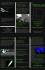### Sea Ray Overnighter 220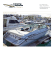### Ray J Crook Obituary web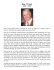### 17/63 George Street, BEENLEIGH, QLD, 4207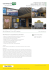### el ray_eng v2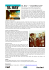### weight loss coaching injury rehabilitation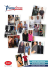### Kemper Lakes Golf Club - A Ray Of Hope On Earth